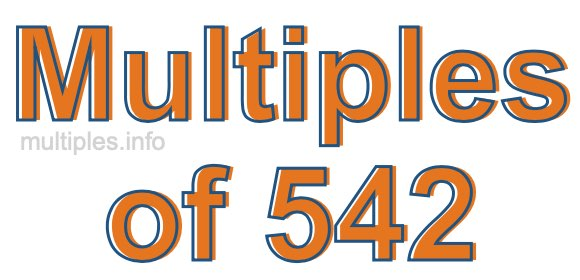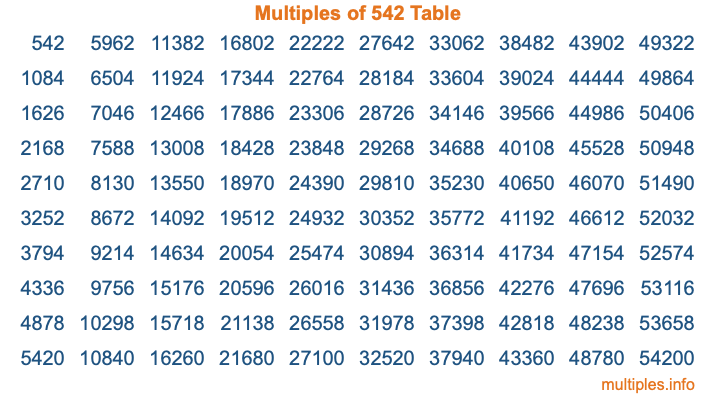Multiples of 542Welcome to the Multiples of 542 page. Here we will first teach you everything you will ever need to know about the multiples of 542, and then give you a study guide summary of everything we taught you to make sure you remember it all. Use this page to look up facts and learn information about the multiples of 542. This page will make you a multiples of five hundred forty-two expert!

Definition of Multiples of 542
Multiples of 542 are all the numbers that when divided by 542 equal an integer. Each of the multiples of 542 are called a multiple. A multiple of 542 is created by multiplying 542 by an integer.

Therefore, to create a list of multiples of 542, you start with 1 multiplied by 542, then 2 multiplied by 542, then 3 multiplied by 542, and so on for as long as you want. Thus, the list of the first five multiples of 542 is 542, 1084, 1626, 2168, and 2710. To see a larger list of multiples of 542, see the printable image of Multiples of 542 further down on this page. We also have a category where you can choose any nth multiple of 542.

Multiples of 542 Checker
The Multiples of 542 Checker below checks to see if any number of your choice is a multiple of 542. In other words, it checks to see if there is any number (integer) that when multiplied by 542 will equal your number. To do that, we divide your number by 542. If the the quotient is an integer, then your number is a multiple of 542.

Is  a multiple of 542?

Least Common Multiple of 542 and ...
A Least Common Multiple (LCM) is the lowest multiple that two or more numbers have in common. This is also called the smallest common multiple or lowest common multiple and is useful to know when you are adding our subtracting fractions. Enter one or more numbers below (542 is already entered) to find the LCM.

Check out our LCM Calculator if you need more details about the Least Common Multiple or if you need the LCM for different numbers for adding and subtraction fractions.

nth Multiple of 542
As we stated above, 542 is the first multiple of 542, 1084 is the second multiple of 542, 1626 is the third multiple of 542, and so on. Enter a number below to find the nth multiple of 542.

th multiple of 542

Multiples of 542 vs Factors of 542
542 is a multiple of 542 and a factor of 542, but that is where the similarities end. All postive multiples of 542 are 542 or greater than 542. All positive factors of 542 are 542 or less than 542.

Below is the beginning list of multiples of 542 and the factors of 542 so you can compare:

Multiples of 542: 542, 1084, 1626, 2168, 2710, etc.

Factors of 542: 1, 2, 271, 542

As you can see, the multiples of 542 are all the numbers that you can divide by 542 to get a whole number. The factors of 542, on the other hand, are all the whole numbers that you can multiply by another whole number to get 542.

It's also interesting to note that if a number (x) is a factor of 542, then 542 will also be a multiple of that number (x).

Multiples of 542 vs Divisors of 542
The divisors of 542 are all the integers that 542 can be divided by evenly. Below is a list of the divisors of 542.

Divisors of 542: 1, 2, 271, 542

The interesting thing to note here is that if you take any multiple of 542 and divide it by a divisor of 542, you will see that the quotient is an integer.

Multiples of 542 Table
Below is an image of the first 100 multiples of 542 in a table. The table is in chronological order, column by column. The first column has the first ten multiples of 542, the second column has the next ten multiples of 542, and so on.The Multiples of 542 Table is also referred to as the 542 Times Table or Times Table of 542. You are welcome to print out our table for your studies.

Negative Multiples of 542
Although not often discussed or needed in math, it is worth mentioning that you can make a list of negative multiples of 542 by multiplying 542 by -1, then by -2, then by -3, and so on, to get the following list of negative multiples of 542:

-542, -1084, -1626, -2168, -2710, etc.

Multiples of 542 Summary
Below is a summary of important Multiples of 542 facts that we have discussed on this page. To retain the knowledge on this page, we recommend that you read through the summary and explain to yourself or a study partner why they hold true.

There are an infinite number of multiples of 542.

A multiple of 542 divided by 542 will equal a whole number.

542 divided by a factor of 542 equals a divisor of 542.

The nth multiple of 542 is n times 542.

The largest factor of 542 is equal to the first positive multiple of 542.

542 is a multiple of every factor of 542.

542 is a multiple of 542.

A multiple of 542 divided by a divisor of 542 equals an integer.

542 divided by a divisor of 542 equals a factor of 542.

Any integer times 542 will equal a multiple of 542.

Multiples of a Number
Here you can get the multiples of another number, all with the same attention to detail as we did for multiples of 542 on this page.

Multiples of
Multiples of 543
Did you find our page about multiples of five hundred forty-two educational? Do you want more knowledge? Check out the multiples of the next number on our list!